# fistree

Network of connected fuzzy inference systems

Since R2019a

## Description

Use a `fistree` object to represent a tree of interconnected fuzzy inference systems.

## Creation

### Syntax

``fisTree = fistree(fis,connections)``
``fisTree = fistree(___,Name,Value)``

### Description

example

````fisTree = fistree(fis,connections)` creates a network of interconnected fuzzy inference system objects, setting its `FIS` and `Connections` properties```
````fisTree = fistree(___,Name,Value)` sets the `Name` or `DisableStructuralChecks` property of the FIS tree using name-value arguments. Specify these arguments after the arguments described in the previous syntax. For example, `fistree(__,'Name',"mytree")` sets the name of the FIS tree to `"mytree"`. You can specify both properties using two name-value arguments.```

## Properties

expand all

FIS tree name, specified as a string or character vector.

Fuzzy inference systems, specified as an array of FIS objects. You can specify any combination of `mamfis`, `sugfis`, `mamfistype2`, and `sugfistype2` objects. Each fuzzy inference system in the `fis` array must have at least one input and one output for `fistree` construction. To evaluate a `fistree`, each fuzzy inference system must have at least one rule.

Connections between fuzzy inference systems, specified as a two-column string array. Each row represents a connection between two FIS objects. You can specify two types of connections.

1. Output-to-input connections — Specify a connection from the output of one FIS to the input of a different FIS.

2. Input-to-input connections — Specify a connection between two inputs so that they use the same input value.

To define a connection, specify the FIS name and variable name for both the source a destination of the connection. For example, ```["fisFrom/out1" "fisTo/in1"]``` defines a connection from output `out1` of FIS `fisFrom` to input `in1` of FIS `fisTo`.

The following figure demonstrates the different connection types using three FIS objects, `fis1`, `fis2`, and `fis3`.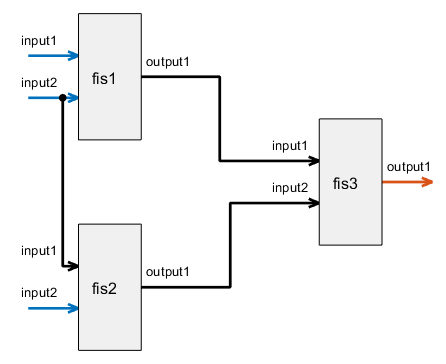This FIS tree includes the following connections.

• `["fis1/output1" "fis3/input1"]` — Connection from output `output1` of `fis1` to input `input1` of `fis3`

• `["fis2/output1" "fis3/input2"]` — Connection from output `output1` of `fis2` to input `input2` of `fis3`

• `["fis1/input2" "fis2/input1"]` — Connection from input `input2` of `fis1` to input `input1` of `fis2`

Connections must satisfy the following conditions:

1. A `fistree` object must have at least one FIS input without any incoming connection and one FIS output without any outgoing connection.

2. A FIS input cannot have more than one incoming connection.

3. A FIS output can have more than one outgoing connection.

4. An input and output of the same FIS cannot be connected. In other words, you cannot create loops between connected FIS objects.

5. Symmetric connections between two inputs are not supported, For example, `["fis1/a" "fis2/b";"fis2/b" "fis1/a"]` is not supported. Instead, specify `["fis1/a" "fis2/b"]` or ```["fis2/b" "fis1/a"]```.

6. Self-input loops, such as `["fis1/a" "fis1/a"]`, are not supported.

Inputs to the FIS tree, specified as an array of strings. Inputs are automatically determined using the specified connections of the `fistree` object. FIS inputs with no incoming connections are included in `Inputs`.

Update this property by updating the connections of the `fistree` object.

Outputs of the FIS tree, specified as a string array. Outputs are automatically determined using the specified connections of the `fistree` object. FIS outputs without any outgoing connections are included in `Outputs`.

You can update this property after initial construction of the `fistree` object. You can remove an existing input or add additional intermediate outputs. `Outputs` must contain at least one output.

Option for disabling structural checks when the FIS tree is updated after initial FIS creation, specified as one of the following values.

• `false` — Automatically update connections, inputs, and outputs. This option ensures that the FIS tree is always valid and can be evaluated.

• `true` — Do not automatically update connections, inputs, and outputs. In this case, the resulting FIS tree can be in an invalid state, which can cause an error during evaluation.

Enable this option to improve computational efficiency when you programmatically construct a FIS tree.

## Object Functions

 `evalfis` Evaluate fuzzy inference system `plotfis` Display fuzzy inference system `getTunableSettings` Obtain tunable settings from fuzzy inference system `getTunableValues` Obtain values of tunable parameters from fuzzy inference system `setTunableValues` Specify tunable parameter values of a fuzzy inference system

## Examples

collapse all

Create a Mamdani fuzzy inference system and a Sugeno fuzzy inference system.

```fis1 = mamfis('Name','fis1','NumInputs',2,'NumOutputs',1); fis2 = sugfis('Name','fis2','NumInputs',2,'NumOutputs',1);```

Define a connection from the output of `fis1` to the first input of `fis2`.

`con1 = ["fis1/output1" "fis2/input1"];`

Create a FIS tree using the fuzzy inference systems and connection.

`tree = fistree([fis1 fis2],con1);`

Visualize the tree.

`plotfis(tree)`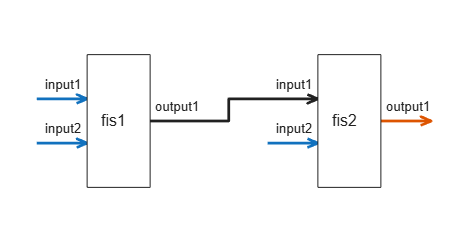Create a tree with two FISs and no connections.

```fis1 = mamfis('Name','fis1','NumInputs',2,'NumOutputs',1); fis2 = mamfis('Name','fis2','NumInputs',2,'NumOutputs',1); fisT = fistree([fis1 fis2],[]);```

Display the FIS tree configuration.

`plotfis(fisT)`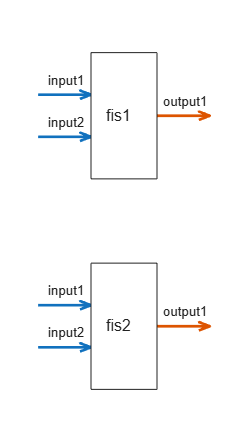You can add a FIS to a FIS tree by appending a FIS object to the FIS property of the tree.

Add `fis3` to the FIS tree.

```fis3 = mamfis('Name','fis3','NumInputs',2,'NumOutputs',1); fisT.FIS(end+1) = fis3;```

Connect the outputs of `fis1` and `fis2` to the inputs of `fis3`.

```fisT.Connections = [ "fis1/output1" "fis3/input1"; "fis2/output1" "fis3/input2"];```

Display the updated FIS tree configuration.

`plotfis(fisT)`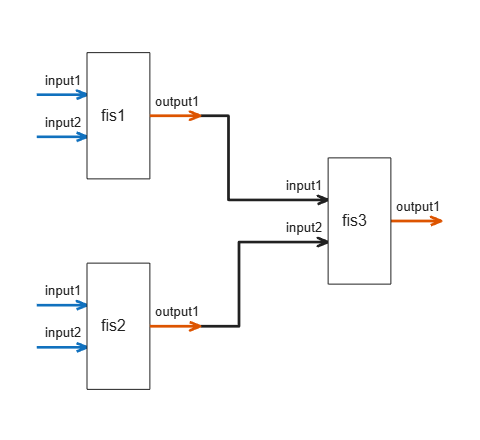Remove the first FIS (`fis1`) from the FIS tree.

`fisT.FIS(1) = [];`

Display the updated FIS tree configuration.

`plotfis(fisT)`The corresponding connection to the first input of `fis3` is also removed.

Create `fis1`, `fis2`, and `fis3`, each with two inputs and one output.

```fis1 = mamfis('Name','fis1','NumInputs',2,'NumOutputs',1); fis2 = mamfis('Name','fis2','NumInputs',2,'NumOutputs',1); fis3 = mamfis('Name','fis3','NumInputs',2,'NumOutputs',1);```

Create a connection between output 1 of `fis1` and input 1 of `fis3`.

`con1 = ["fis1/output1" "fis3/input1"];`

Create a connection between output 1 of `fis2` and input 2 of `fis3`.

`con2 = ["fis2/output1" "fis3/input2"];`

Create a connection between input 2 of `fis1` and input 1 of `fis2`.

`con3 = ["fis1/input2" "fis2/input1"];`

Create and display the FIS tree.

```fuzzTree = fistree([fis1 fis2 fis3],[con1;con2;con3]); plotfis(fuzzTree)```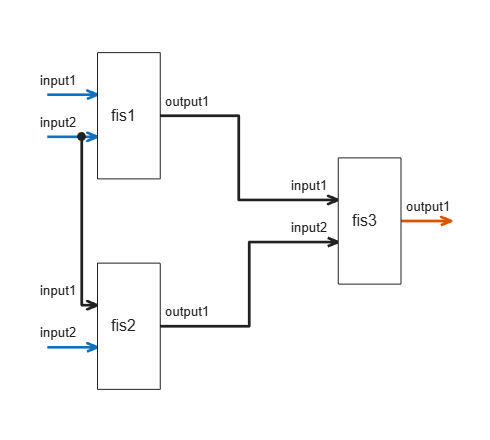Display the inputs of the FIS tree. These inputs correspond to all free inputs that do not have an incoming connection.

`fuzzTree.Inputs`
```ans = 3x1 string "fis1/input1" "fis1/input2" "fis2/input2" ```

Evaluate the fuzzy tree. Specify values for input 1 of `fis1`, input 2 of `fis1`, and input 2 of `fis2`. The value for input 2 of `fis1` is also applied to input 1 of `fis2`.

`output = evalfis(fuzzTree,[0.8 0.25 0.7]);`

Create two FIS objects, each with two inputs and one output.

```fis1 = mamfis('Name','fis1','NumInputs',2,'NumOutputs',1); fis2 = mamfis('Name','fis2','NumInputs',2,'NumOutputs',1);```

Connect the output of `fis1` to the second input of `fis2`.

`con = ["fis1/output1" "fis2/input2"];`

Create the FIS tree.

`fuzzTree = fistree([fis1 fis2],con);`

Display outputs of the FIS tree. By default, the open FIS output from `fis2` is an output of the FIS tree.

`fuzzTree.Outputs`
```ans = "fis2/output1" ```

Visualize the FIS tree.

`plotfis(fuzzTree,Legend="on")`Add the output of `fis1` to the list of outputs.

`fuzzTree.Outputs(end+1) = "fis1/output1";`

Display the updated output list of the FIS tree.

`fuzzTree.Outputs`
```ans = 2x1 string "fis2/output1" "fis1/output1" ```

Visualize the FIS tree. The added intermediate output is highlighted.

`plotfis(fuzzTree,Legend="on")`Evaluate the FIS tree. The result contains the outputs from `fis2` and `fis1`.

`evalfis(fuzzTree,[0.5 0.2 0.8])`
```ans = 1×2 0.1579 0.1579 ```

Remove the first output from the list.

`fuzzTree.Outputs(1) = [];`

Display the updated output list of the FIS tree.

`fuzzTree.Outputs`
```ans = "fis1/output1" ```

Visualize the FIS tree without the removed output. The visualization indicates that the output of `fis2` is unused.

`plotfis(fuzzTree,Legend="on")`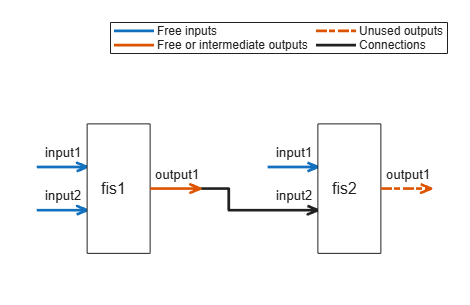Evaluate the FIS tree again. The result now contains the output of only `fis2`.

`evalfis(fuzzTree,[0.5 0.2 0.8])`
```ans = 0.1579 ```

This example shows the construction of an incremental FIS tree. For more information on the types of fuzzy tree structures, see Fuzzy Trees.

Create fuzzy systems `fis1`, `fis2`, and `fis3`, each with two inputs and one output.

```fis1 = mamfis('Name','fis1','NumInputs',2,'NumOutputs',1); fis1.Inputs(1).Name = "color"; fis1.Inputs(2).Name = "doors"; fis2 = mamfis('Name','fis2','NumInputs',2,'NumOutputs',1); fis2.Inputs(2).Name = "power"; fis3 = mamfis('Name','fis3','NumInputs',2,'NumOutputs',1); fis3.Inputs(2).Name = "autopilot"; fis3.Outputs(1).Name = "prediction";```

Create a connection between output 1 of `fis1` and input 1 of `fis2`.

`con1 = ["fis1/output1" "fis2/input1"];`

Create a connection between output 1 of `fis2` and input 1 of `fis3`.

`con2 = ["fis2/output1" "fis3/input1"];`

Create the FIS tree.

`incTree = fistree([fis1 fis2 fis3],[con1;con2]);`

Visualize the tree structure. At each level of the tree structure, an additional input is combined with the output of the previous level.

`plotfis(incTree)`This example shows the construction of an aggregated FIS tree. For more information on the types of fuzzy tree structures, see Fuzzy Trees.

Create fuzzy systems `fis1`, `fis2`, and `fis3`, each with two inputs and one output.

```fis1 = mamfis('Name','fis1','NumInputs',2,'NumOutputs',1); fis1.Inputs(1).Name = "dist_obs"; fis1.Inputs(2).Name = "angle_obs"; fis2 = mamfis('Name','fis2','NumInputs',2,'NumOutputs',1); fis2.Inputs(1).Name = "dist_tar"; fis2.Inputs(2).Name = "angle_tar"; fis3 = mamfis('Name','fis3','NumInputs',2,'NumOutputs',1); fis3.Outputs(1).Name = "heading_robot";```

Create a connection between output 1 of `fis1` and input 1 of `fis3`.

`con1 = ["fis1/output1" "fis3/input1"];`

Create a connection between output 1 of `fis2` and input 2 of `fis3`.

`con2 = ["fis2/output1" "fis3/input2"];`

Create the FIS tree.

`aggTree = fistree([fis1 fis2 fis3],[con1;con2]);`

Visualize the tree structure.

`plotfis(aggTree)`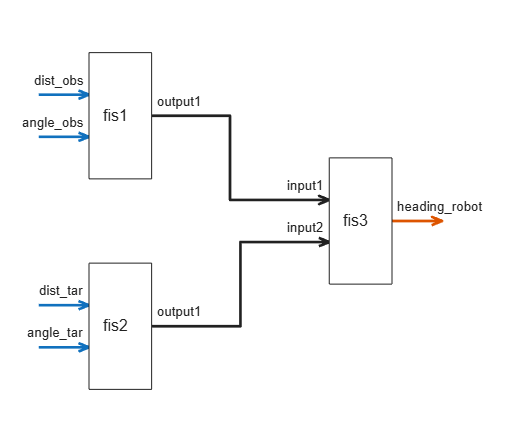This example shows the construction of a cascaded FIS tree. For more information on the types of fuzzy tree structures, see Fuzzy Trees.

Create fuzzy systems `fis1`, `fis2`, `fis3`, and `fis4`, each with two inputs and one output.

```fis1 = mamfis('Name','fis1','NumInputs',2,'NumOutputs',1); fis1.Inputs(1).Name = "dist_obs"; fis1.Inputs(2).Name = "angle_obs"; fis2 = mamfis('Name','fis2','NumInputs',2,'NumOutputs',1); fis2.Inputs(1).Name = "dist_tar"; fis2.Inputs(2).Name = "angle_tar"; fis3 = mamfis('Name','fis3','NumInputs',2,'NumOutputs',1); fis4 = mamfis('Name','fis4','NumInputs',2,'NumOutputs',1); fis4.Inputs(2).Name = "preheading_robot"; fis4.Outputs(1).Name = "heading_robot";```

Create a connection between output 1 of `fis1` and input 1 of `fis3`.

`con1 = ["fis1/output1" "fis3/input1"];`

Create a connection between output 1 of `fis2` and input 2 of `fis3`.

`con2 = ["fis2/output1" "fis3/input2"];`

Create a connection between output 1 of `fis3` and input 1 of `fis4`.

`con3 = ["fis3/output1" "fis4/input1"];`

Create the FIS tree.

`casTree = fistree([fis1 fis2 fis3 fis4],[con1;con2;con3]);`

Visualize the tree structure.

`plotfis(casTree)`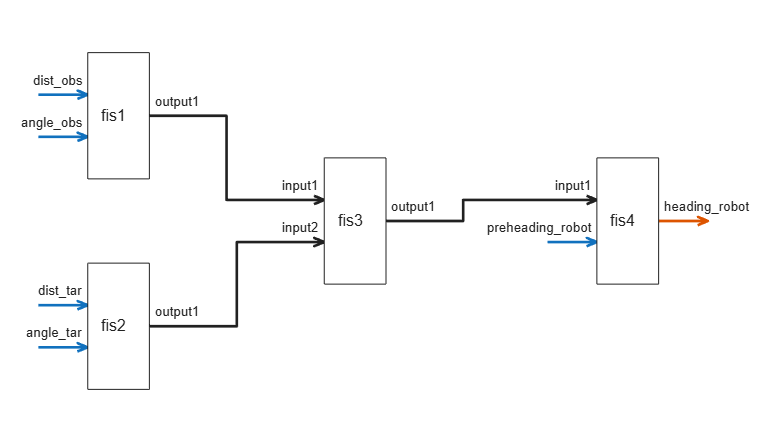This example shows the construction of a parallel FIS tree. For more information on the types of fuzzy tree structures, see Fuzzy Trees.

Create fuzzy systems `fis1`, `fis2`, and `fis3`, each with two inputs and one output.

```fis1 = mamfis('Name','fis1','NumInputs',2,'NumOutputs',1); fis2 = mamfis('Name','fis2','NumInputs',2,'NumOutputs',1);```

Create the FIS tree such that the FIS objects are in parallel; that is, the tree contains no interconnections and all the FIS outputs are FIS tree outputs.

`parTree = fistree([fis1 fis2],[]);`

Visualize the tree.

`plotfis(parTree)`Evaluate the FIS tree.

`output = evalfis(parTree,[0.1 0.3 0.8 0.4]);`

Generate the final output by summing the FIS tree outputs.

`finalOutput = sum(output);`

## Version History

Introduced in R2019a

expand all